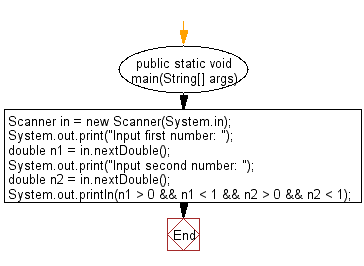﻿ Java programming exercises: Accepts two double variables and test the specified condition - w3resource# Java Exercises: Accepts two double variables and test the specified condition

## Java Basic: Exercise-153 with Solution

Write a Java program that accepts two double variables and test if both strictly between 0 and 1 and false otherwise.

Sample Solution:

Java Code:

``````import java.util.Scanner;
public class Solution {
public static void main(String[] args) {
Scanner in = new Scanner(System.in);
System.out.print("Input first number: ");
double n1 = in.nextDouble();
System.out.print("Input second number: ");
double n2 = in.nextDouble();
System.out.println(n1 > 0 && n1 < 1 && n2 > 0 && n2 < 1);
}
}
```
```

Sample Output:

```(101 + 0) / 3)-> 33
(3.0e-6 * 10000000.1)-> 30.0000003
(true && true)-> true
(false && true)-> false
((false && false) || (true && true))-> true
(false || false) && (true && true)-> false
```

Flowchart:Java Code Editor:

What is the difficulty level of this exercise?

﻿

## Java: Tips of the Day

Parsing dates:

```import java.io.*;
import java.util.*;
import java.text.*;

String s = "2001/09/23 14:39";

SimpleDateFormat formatter = new SimpleDateFormat ("yyyy/MM/dd H:mm");
Date d = formatter.parse(s, new ParsePosition(0));
```